# Goursat problem

The Goursat problem concerns a hyperbolic partial differential equation, or a second-order hyperbolic system, in two independent variables with given values on two characteristic curves emanating from the same point.

For the hyperbolic equation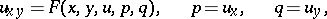(1)

given, for example, in the domain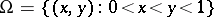, Goursat's problem is posed as follows: To find a solutionof (1) that is regular inand continuous in the closurefrom the boundary conditions(2)

whereandare given continuously-differentiable functions. Ifis continuous for all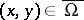and any system of real values of the variables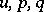and if it has derivatives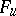,andthe absolute value of which under these conditions is smaller than a certain number, then a unique and stable solution of the problem (1), (2) exists in.

In the study of the linear case of Goursat's problem,(3)

a fundamental role is played by the Riemann function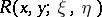, which is uniquely defined as the solution of the equation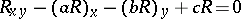that, on the characteristics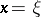and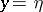, satisfies the condition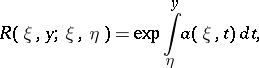where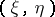is an arbitrary point in the domainin which equation (3) is defined. If the functions,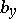andare continuous, then the Riemann function exists and is, with respect to the variablesand, the solution of the equation.

The solution of Goursat's problem (2) for equation (3) is given by the so-called Riemann formula. If, it has the form: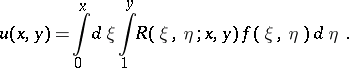It follows from Riemann's formula that the valueof the solution of Goursat's problem at a pointdepends only on the value of the given functions in the characteristic quadrilateral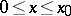,. If, this value depends only on the values of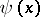and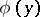in the intervalsand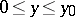, respectively, while if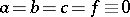, the function has the form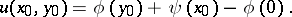The method of obtaining explicit formulas for the solution of Goursat's problem using the Riemann function is known as the Riemann method. The method has been extended to a fairly wide class of hyperbolic systems of orders one and two — in particular, to systems of the form (3) where,andare quadratic symmetric matrices of order, whileandare vectors withcomponents.

A direct generalization of Goursat's problem is the Darboux–Picard problem: To find the solution of a hyperbolic equation, or a second-order hyperbolic system, in two independent variables from its given values on two smooth monotone curvesand, issuing from the same pointand located in the characteristic angle with apex at. In particular,andmay partly or wholly coincide with the sides of this angle. This problem has been studied for equations of the form (1).

Goursat's problem is sometimes referred to as the Darboux problem. The Goursat problem for hyperbolic equations of order two in several independent variables is often understood to be the characteristic problem, viz. to find its solution from given values on the characteristic conoid (cf. Differential equation, partial, data on characteristics).

The problem is named after E. Goursat, who studied it in detail.